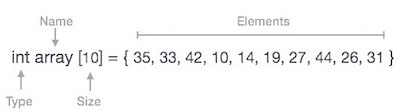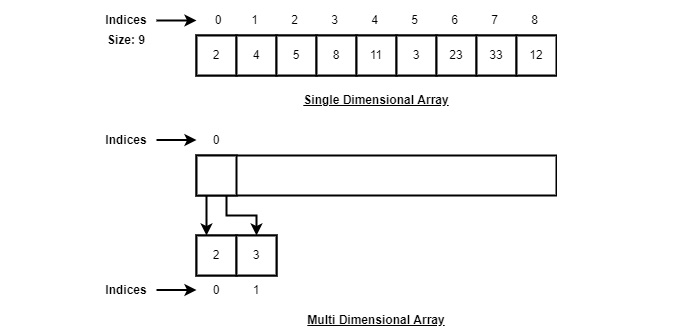# Python - Arrays

Array is a container which can hold a fix number of items and these items should be of the same type. Most of the data structures make use of arrays to implement their algorithms. Following are the important terms to understand the concept of Array.

• Element− Each item stored in an array is called an element.
• Index − Each location of an element in an array has a numerical index, which is used to identify the element.

## Array Representation

Arrays can be declared in various ways in different languages. Below is an illustration.As per the above illustration, following are the important points to be considered.

• Index starts with 0.

• Array length is 10 which means it can store 10 elements.

• Each element can be accessed via its index. For example, we can fetch an element at index 6 as 9.

## Basic Operations

Following are the basic operations supported by an array.

• Traverse − print all the array elements one by one.

• Insertion − Adds an element at the given index.

• Deletion − Deletes an element at the given index.

• Search − Searches an element using the given index or by the value.

• Update − Updates an element at the given index.

Array is created in Python by importing array module to the python program. Then the array is declared as shown eblow.

```
from array import *

arrayName = array(typecode, [Initializers])
```

Typecode are the codes that are used to define the type of value the array will hold. Some common typecodes used are:

Typecode Value
b Represents signed integer of size 1 byte/td>
B Represents unsigned integer of size 1 byte
c Represents character of size 1 byte
i Represents signed integer of size 2 bytes
I Represents unsigned integer of size 2 bytes
f Represents floating point of size 4 bytes
d Represents floating point of size 8 bytes

Before lookign at various array operations lets create and print an array using python.

The below code creates an array named array1.

```from array import *

array1 = array('i', [10,20,30,40,50])

for x in array1:
print(x)
```

When we compile and execute the above program, it produces the following result −

```
10
20
30
40
50

```

## Accessing Array Element

We can access each element of an array using the index of the element. The below code shows how

```
from array import *

array1 = array('i', [10,20,30,40,50])

print (array1)

print (array1)
```

When we compile and execute the above program, it produces the following result − which shows the element is inserted at index position 1.

```
10
30

```

## Insertion Operation

Insert operation is to insert one or more data elements into an array. Based on the requirement, a new element can be added at the beginning, end, or any given index of array.

Here, we add a data element at the middle of the array using the python in-built insert() method.

```
from array import *

array1 = array('i', [10,20,30,40,50])

array1.insert(1,60)

for x in array1:
print(x)
```

When we compile and execute the above program, it produces the following result which shows the element is inserted at index position 1.

```
10
60
20
30
40
50

```

## Deletion Operation

Deletion refers to removing an existing element from the array and re-organizing all elements of an array.

Here, we remove a data element at the middle of the array using the python in-built remove() method.

```
from array import *

array1 = array('i', [10,20,30,40,50])

array1.remove(40)

for x in array1:
print(x)
```

When we compile and execute the above program, it produces the following result which shows the element is removed form the array.

```10
20
30
50
```

## Search Operation

You can perform a search for an array element based on its value or its index.

Here, we search a data element using the python in-built index() method.

```
from array import *

array1 = array('i', [10,20,30,40,50])

print (array1.index(40))

```

When we compile and execute the above program, it produces the following result which shows the index of the element. If the value is not present in the array then th eprogram returns an error.

```3
```

## Update Operation

Update operation refers to updating an existing element from the array at a given index.

Here, we simply reassign a new value to the desired index we want to update.

```
from array import *

array1 = array('i', [10,20,30,40,50])

array1 = 80

for x in array1:
print(x)

```

When we compile and execute the above program, it produces the following result which shows the new value at the index position 2.

```10
20
80
40
50
```